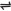## How to Determine Strength of Acids

The strength of acids is dependent upon:

1. Their willingness to furnish hydronium ion, H3O+ in solution.

2. The number of hydronuim ions per mole they are willing to furnish. On the above basis, acids with high readiness to produce H3O+, as well as completely ionizing are regarded as been strong. Others, with low readiness to ionize and which undergo partial ionization are regarded as weak acids.

Thus, we may compare the acidities of acids of moderate strengths by comparing their ionization constants. Ionization constants are equilibrium constants for the reactions of the acids with water. The smaller the ionization constant, the weaker is the acid, and vice versa.

Example, consider the ionization constant of the following acids at 25oC.

Tetraoxosulphate(VI) acid:

 H2SO4(aq) + 2H2O(l)2H3O+(aq) + SO42-(aq) complete ionization K = appro. 1

Hydrochloric acid:

 HCl(aq) + H2O(l)H3O+(aq) + Cl-(aq) complete ionization K = appro. 1

Tetraoxophosphate(V) acid:

 H3PO4(aq) + H2O(l)H3O+(aq) + H2PO4-(aq) K = 7.5 x 10-3

Acetic (ethanoic) acid:

 CH3COOH(aq) + H2O(l)H3O+(aq) + CH3COO-(aq) K = 1.8  x 10-5

By comparing the ionization constants of the above acids, we perceive immediately that the order of strength is

H2SO4(aq) > HCl(aq )> H3PO4(aq) > CH3COOH(aq)

Explanation: Even though H3PO4(aq) can eventually produce 3 molecules of H3O+, it does not readily ionize, compared with HCl(aq) which produces 1 molecule of H3O+ - HCl can readily and completely ionize. H2SO4(aq), readily and completely ionizes to produce 2 molecules of H3O+, while CH3COOH(aq) does not ionize readily and completely, infact, out of four atoms of hydrogen contained per molecule, it can only ionize one - hence, it is the least in strength amongst the given acids.

Note: the strength of acids does not depend on their basicity. This is because basicity does not consider the ability of the acid to ionize. Strong Acid: H2SO4, HCl and HNO3. Weak Acid: H2CO3, H3PO4, CH3COOH and all organic acids.

 Like This Post? Please Share!!

Copyright , All Rights Reserved Free Chemistry Online | About Us | Usage of Content | Total Disclosures | Privacy Policy